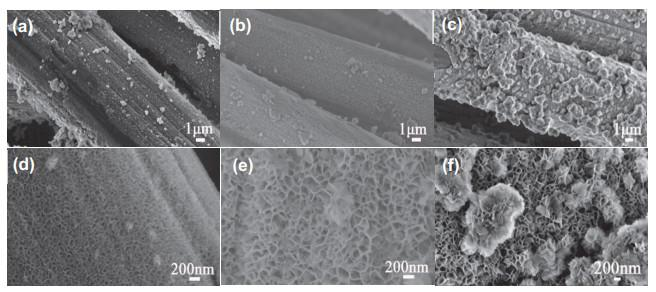## Synthesis and Capacitive Property Analysis of a Nickel Hydroxide Electrode Material Located on Carbon Cloth: A Recommended Comprehensive Chemistry Experiment

Zhang Lin,, Yao Hongchang, Li Zhaohui, Li Zhongjun

 基金资助: 郑州大学2020年度校级教育教学改革研究与实践项目.  2020zzuJXLX175郑州大学2020年度校级教育教学改革研究与实践项目.  2020zzuJXLX022

Received: 2021-08-23   Accepted: 2021-10-8Abstract

Ni(OH)2 has many advantages as an electrode material for supercapacitors, including a high theoretical specific capacitance, abundant sources, and that it is environmentally friendly. However, its low conductivity significantly limits its electrochemical properties. An effective method to address this issue is through the in situ growth of Ni(OH)2 on a conductive carbon cloth substrate. Herein, we propose a comprehensive chemical experiment that includes the synthesis of Ni(OH)2 on carbon cloth, characterization of the electrode materials, and determination of its electrochemical properties. Such a comprehensive experiment will strengthen students' understanding of the chemical equilibrium principle applied in material synthesis, expose them to additional characterization methods and advanced electrochemical analysis methods, such as cyclic voltammetry and galvanostatic charging and discharging, establish basic theoretical knowledge of electrochemistry, and enhance their laboratory skills and comprehensive ability. In addition, this experiment can also assist students to understand the frontier of energy storage technology, stimulate their interest in scientific research, and cultivate their awareness of scientific research innovations. Therefore, this experiment is suitable for use as a college chemistry experiment.

Keywords： Nickel hydroxide ; Supercapacitor ; Cyclic voltammetry ; Galvanostatic charge/discharge ; Comprehensive experiment

Zhang Lin. Synthesis and Capacitive Property Analysis of a Nickel Hydroxide Electrode Material Located on Carbon Cloth: A Recommended Comprehensive Chemistry Experiment. University Chemistry[J], 2022, 37(2): 2108063-0 doi:10.3866/PKU.DXHX202108063

## 1 实验目的

(1) 了解CBD法的合成方法和成膜机理；

(2) 了解X射线衍射仪的基本操作，掌握物相结构分析方法；

(3) 了解扫描电镜的测试操作，掌握形貌分析方法；

(4) 了解循环伏安法、直流充放电法的基本原理和实验操作方法；

(5) 掌握电容性能的分析方法。

### 2.1 CBD法成膜机理

CBD法是将基底浸入含有金属离子、配合剂和阴离子的前驱溶液中，常压、常温下制备薄膜的一种液相化学方法。CBD法制备Ni(OH)2薄膜涉及的化学反应如下所示

${\rm{N}}{{\rm{i}}^{{\rm{2 + }}}}{\rm{(aq) + }}x{\rm{N}}{{\rm{H}}_{\rm{3}}}{\rm{(aq) + }}\left( {{\rm{6 - x}}} \right){{\rm{H}}_{\rm{2}}}{\rm{O(l)}} \leftrightarrow {\left[ {{\rm{Ni}}{{\left( {{{\rm{H}}_{\rm{2}}}{\rm{O}}} \right)}_{{\rm{6 - }}x}}{{\left( {{\rm{N}}{{\rm{H}}_{\rm{3}}}} \right)}_x}} \right]^{{\rm{2 + }}}}{\rm{(aq)}}$

${\rm{N}}{{\rm{H}}_{\rm{3}}} \cdot {{\rm{H}}_{\rm{2}}}{\rm{O(l)}} \leftrightarrow {\rm{NH}}_{\rm{4}}^{\rm{ + }}{\rm{(aq) + O}}{{\rm{H}}^{\rm{ - }}}{\rm{(aq)}}$

${{\rm{[Ni(}}{{\rm{H}}_{\rm{2}}}{\rm{O}}{{\rm{)}}_{{\rm{6 - }}x}}{{\rm{(N}}{{\rm{H}}_{\rm{3}}}{\rm{)}}_x}{\rm{]}}^{{\rm{2 + }}}}{\rm{(aq) + 2O}}{{\rm{H}}^ - }{\rm{(aq)}} \leftrightarrow {\rm{Ni(OH}}{{\rm{)}}_{\rm{2}}}{\rm{(s) + (6 - }}x{\rm{)}}{{\rm{H}}_{\rm{2}}}{\rm{O(l) + }}x{\rm{N}}{{\rm{H}}_{\rm{3}}}{\rm{(aq)}}$

${\rm{2Ni(OH}}{{\rm{)}}_{\rm{2}}}{\rm{(s) + }}{{\rm{S}}_{\rm{2}}}{\rm{O}}_{\rm{8}}^{{\rm{2 - }}}{\rm{(aq) + 2O}}{{\rm{H}}^ - }{\rm{(aq) }} \to {\rm{2NiOOH(s) + 2SO}}_{\rm{4}}^{{\rm{2 - }}}{\rm{(aq) + 2}}{{\rm{H}}_{\rm{2}}}{\rm{O(l)}}$

${\rm{ 6NiOOH(s) + 2N}}{{\rm{H}}_{\rm{3}}} \cdot {{\rm{H}}_{\rm{2}}}{\rm{O(l) }} \to {\rm{6Ni}}{\left( {{\rm{OH}}} \right)_2}\left( {\rm{s}} \right) + {{\rm{H}}_2}{\rm{O}}\left( {\rm{g}} \right) + {{\rm{N}}_{\rm{2}}}\left( {\rm{g}} \right)$

### 图1### 2.3 循环伏安法(Cyclic voltammetry，CV) 

CV测试是一种常用的电化学方法，该法控制电极电势以三角波形反复扫描，得到电流–电势曲线。在扫描的某些电位区间会出现波峰，表明在该电位区间进行着某些电化学反应或表面吸附。根据CV曲线的形状可以判断电极材料储能的类型以及可逆程度。对于完全可逆的电极表面过程，CV曲线上氧化电位(EPa)和还原电位(EPc)值相等，即EPaEPc = ΔEP = 0，峰电位与扫描速度(v)无关，峰电流与v的一次方成正比，且氧化电流(IPa)和还原电流(IPc)相等，即IPa/IPc = 1；对于电极反应不完全可逆(比如准可逆或不可逆)，其ΔEP ≠ 0，IPa/IPc ≠ 1，差别程度由电极反应的可逆性决定。

CV法还可以用于分析电极材料的电荷储存动力学。在不同的v下进行扫描，得到不同的IP。二者之间有如下的关系：

${I_{\rm{P}}}{\rm{ = }}a{v^b}{\kern 1pt} {\kern 1pt} {\kern 1pt} {\kern 1pt} {\kern 1pt} {\kern 1pt} {\kern 1pt} {\kern 1pt} {\kern 1pt} {\kern 1pt} {\kern 1pt} {\kern 1pt} {\kern 1pt} {\kern 1pt} 或{\kern 1pt} {\kern 1pt} {\kern 1pt} {\kern 1pt} {\kern 1pt} {\kern 1pt} {\kern 1pt} {\kern 1pt} {\kern 1pt} {\kern 1pt} {\kern 1pt} {\kern 1pt} {\kern 1pt} {\kern 1pt} {\rm{log}}{I_{\rm{P}}}{\rm{ = }}\log a{\rm{ + }}b\log v$

${C_{\text{S}}}{\text{ = }}\frac{1}{{2mv({V_2} - {V_1})}}\int_{{V_1}}^{{V_2}} {IdV}$

### 图2### 2.4 恒流充放电法(Galvanostatic charge and discharge，GCD) 

GCD法又叫计时电位法，在恒流条件下对被测电极进行充放电，记录电位随时间的变化曲线，进而研究电极的充放电性能。恒流充放电测试是评价超级电容器比容量最常用的方法之一。在实验中设定不同的电流密度，根据恒流放电曲线数据，可以计算出不同电流密度下的比电容。具体公式如下：

${C_{\rm{s}}}{\rm{ = }}\frac{{I \times \Delta t}}{{m \times \Delta V}}$

CsI，Δt，ΔV分别代表比电容(F·g−1)，恒定的放电电流(A)，放电时间(s)和放电电位窗口(V)。

### 4.3 X射线衍射(XRD)

XRD利用X射线在晶体中的衍射现象获得衍射图谱，通过图谱信息确定材料的物相以及晶体结构。晶体都具有自身特有的化学组成和晶体结构，对X射线的衍射会产生各自特有的衍射花样。粉末衍射标准委员会收集了大量晶体的标准衍射数据，编制成X射线粉末衍射数据卡片(JCPDS卡片)。通过将测得物质粉末的衍射数据与标准JCPDS卡进行对照，可以得到被测物质的化学组成以及相关的各种晶体学数据。晶体物质的衍射面间距d和衍射线相对强度I/I1是晶体结构的特征反映。d与晶胞大小及形状有关，I/I1与晶胞中所含质点的种类、数目以及在晶胞中的位置有关。XRD测试样品的制备方法：取一定量的待测样品粉末放入特制玻璃片的槽内并刮平，然后将玻璃片放入仪器进行测试。实验测试时，使用铜靶作为辐射源，波长为0.15418 nm，扫描范围为5°–80°，扫描速率为5° min−1

### 4.4 扫描电子显微镜(SEM)

SEM是观察样品微观形貌最直观的手段之一。利用电子束与被测样品表面的相互作用，它将产生的电子信息收集、转换得到反映样品表面形貌的SEM图。SEM测试样品的制备方法：首先取少量待测样品涂到导电胶带上，然后对样品进行喷金处理。

## 4.5.2 GCD法

### 图3### 图4(a、d) 5 min；(b、e) 10 min；(c、f) 60 min

#### 5.3.1 CV分析

$\text{Ni}{\left(\text{OH}\right)}_{\text{2}}\text{}\text{+}{\text{}\text{OH}}^{\text{-}}\text{}\text{↔}\text{}\text{NiOOH}\text{}\text{+}\text{}{\text{H}}_{\text{2}}\text{O}$

### 图5### 图6## 6 思考题

1) 在Ni(OH)2制备中，氨水量对反应物的结构将会有什么影响？

2) 过硫酸钾在合成氢氧化镍过程中的作用？

3) 氢氧化镍的储能机理是什么？它与双电层电容材料的电化学性质有何区别？

## 参考文献 原文顺序 文献年度倒序 文中引用次数倒序 被引期刊影响因子

Jing L. ; Zhang L. ; Li G. ; Guo Z. ; Li Z. ; Yao H. ; Wang J. ; Liu Q. J. Alloy Compd. 2021, 854, 157246.

Sun J. ; Lu J. ; Li B. ; Jiang L. ; Chesman A. S. R. ; Scully A. D. ; Gengenbach T. R. ; Cheng Y. B. ; Jasieniak J. J. Nano Energy 2018, 49, 163.

/

 〈〉